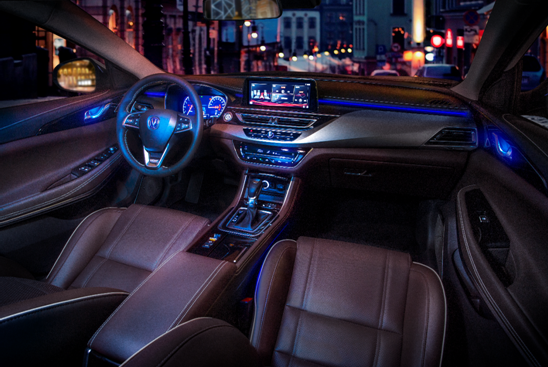## 更个性、更贴心，睿骋CC给你所想

2018年07月19日 15:50 来源：车行天下 超过：10484次关注在移动互联网时代的今天，“智能出行“一直是当下最推崇的出行模式，有没有一款车的出现，可以让更多的人享受到科技带来的便捷与乐趣呢？作为中国品牌领导者的长安睿骋CC便是他们的答案。为了打造更懂消费者的汽车，睿骋CC针对用户最关心的人工智能语音、个性化大数据导航、智慧出行娱乐等方面进行全面的升级，结合全时在线，给用户带来更贴心、更智能的驾控体验。长安汽车基于多年经验积累，从交互需求的变化，不断摸索，延展向更大的交互空间，通过打造个性化、定制化的服务、满足车主越来越多样的需求。升级后的睿骋CC新增了多项个性化功能，如组队出行功能，远途出行时让你告别寂寞乏味，可以呼朋唤友，一起去旅行，劲享智能便捷。此外，为保证用户的行车安全，升级后的睿骋CC还增加了行程分享功能，通过打通车机和微信，用户绑定到微信后，便可以将好友在微信中选择并分享的位置，发送到车机。导航过程中，增加报平安功能，用户可以将自己的实时位置、预计到达时间分享给自己的亲友，让家人更安心。提供了通过短信进行分享，链接蓝牙通讯录后可以直接从通讯录选择，并且可以通过语音直接进行分享。同时，用户还可以通过微信扫码将位置分享给好友。借助阿里巴巴的生态资源，高德地图车机版还增加了智慧停车服务，车主登陆账号，输入车牌号后就可以正式开通该功能。在合作停车场内，车主在出场前进入导航，导航就会对此次的消费情况进行显示，并可通过支付宝在线支付，快速出场，不必担心堵车的烦恼。与升级前相比，新版本版本将更注重与“主人”的友好互动。比如长安in-call中新增语音小精灵功能，可进行提醒车门是否安全关闭、行车过程中急刹车提醒，除此之外，系统可以每天第一时间为您送上问候，还会在专属的节日及生日祝福，当有紧急天气时还会进行告知提箱，让您在每一次出行都能感受到TA给你带来的惊喜。与此同时，还新增了酷我音乐、喜马拉雅等一系列“人无我有”的娱乐功能。此外，“车机可接连手机WIFI，流量共享”功能可以让手机不欠费，流量不断流，这让“基础流量、基础服务终身免费”的升级版睿骋CC，用起来更加爽。(长安汽车美国底特律智能化研究部)

“人无远虑，必有近忧！”长安在智能汽车的全球研发布局方面提前做了大量工作，这也是他们能永远提前半步的原因所在。联合阿里巴巴、因特尔、科大讯飞等公司进行信息化与工业化的高度融合与强强联手。持续在智能车联网平台、车联网服务以及企业社交领域三大方面展开战略合作，为用户提供极致的车联网服务。得益于这些合作，长安in-call智能车载互联系统如今已经迭代到新版本，它集成了收音机、多媒体、电话、导航、语音控制、智慧停车、行程分享、个性化设置及其自动调节等多项功能。这些丰富的车联网功能让睿骋CC的使用体验再上了一个台阶。未来，睿骋CC将在智能系统不断创新，通过智能化的语音控制系统配合个性化的汽车性能，持续改变车主交互方式的同时，丰富车主的驾乘生活，给车主最佳的行车体验。为汽车行业的发展与变革注入更多动力，助力更多汽车品牌从汽车助力智能化水平上更胜一筹，为汽车智能化发展贡献更多力量。

#### 相关文章

0-500 字已有评论 0条 查看评论>>

### 热门标签

﻿
• 快速找车
• 选择品牌
• 选择品牌
• A  奥迪
• A  阿斯顿·马丁
• A  阿尔法·罗密欧
• B  宝沃
• B  布加迪
• B  巴博斯
• B  保时捷
• B  宾利
• B  奔驰
• B  宝马
• B  本田
• B  别克
• B  标致
• B  比亚迪
• B  宝骏
• B  北汽制造
• B  北汽新能源
• B  北汽幻速
• B  北汽威旺
• B  北京汽车
• B  奔腾
• B  北汽绅宝
• C  长安
• C  长安商用
• C  长城
• C  昌河
• D  大众
• D  道奇
• D  DS
• D  东南
• D  东风风神
• D  东风风行
• D  东风小康
• D  东风风度
• D  东风
• F  福特
• F  丰田
• F  菲亚特
• F  法拉利
• F  福田
• F  福迪
• F  福汽启腾
• G  观致
• G  广汽传祺
• G  广汽吉奥
• G  GMC
• H  红旗
• H  汉腾汽车
• H  哈弗
• H  哈飞
• H  海格
• H  海马
• H  华颂
• H  黄海
• H  华泰
• H  恒天
• J  吉利汽车
• J  捷豹
• J  Jeep
• J  江淮
• J  江铃
• J  金杯
• J  九龙
• J  金旅
• K  凯翼
• K  凯迪拉克
• K  克莱斯勒
• K  科尼塞克
• K  卡威
• K  开瑞
• L  路虎
• L  林肯
• L  劳斯莱斯
• L  兰博基尼
• L  雷克萨斯
• L  铃木
• L  雷诺
• L  理念
• L  力帆
• L  莲花汽车
• L  猎豹
• L  路特斯
• L  陆风
• M  马自达
• M  MG
• M  MINI
• M  玛莎拉蒂
• M  摩根
• M  迈凯轮
• N  纳智捷
• O  欧宝
• O  讴歌
• O  欧朗
• Q  奇瑞
• Q  起亚
• Q  启辰
• R  日产
• R  荣威
• R  瑞麒
• S  三菱
• S  斯威汽车
• S  萨博
• S  smart
• S  斯柯达
• S  斯巴鲁
• S  思铭
• S  双龙
• S  上汽大通
• S  双环
• T  特斯拉
• T  腾势
• W  沃尔沃
• W  五菱汽车
• W  五十铃
• W  威兹曼
• W  威麟
• X  现代
• X  雪佛兰
• X  雪铁龙
• X  西雅特
• Y  一汽
• Y  英菲尼迪
• Y  英致
• Y  依维柯
• Y  野马汽车
• Y  永源
• Z  众泰
• Z  中华
• Z  中兴
• Z  知豆
• 选择车系
• 选择车系
• 车型对比
• 选择品牌
• 选择品牌
• A  奥迪
• A  阿斯顿·马丁
• A  阿尔法·罗密欧
• B  宝沃
• B  布加迪
• B  巴博斯
• B  保时捷
• B  宾利
• B  奔驰
• B  宝马
• B  本田
• B  别克
• B  标致
• B  比亚迪
• B  宝骏
• B  北汽制造
• B  北汽新能源
• B  北汽幻速
• B  北汽威旺
• B  北京汽车
• B  奔腾
• B  北汽绅宝
• C  长安
• C  长安商用
• C  长城
• C  昌河
• D  大众
• D  道奇
• D  DS
• D  东南
• D  东风风神
• D  东风风行
• D  东风小康
• D  东风风度
• D  东风
• F  福特
• F  丰田
• F  菲亚特
• F  法拉利
• F  福田
• F  福迪
• F  福汽启腾
• G  观致
• G  广汽传祺
• G  广汽吉奥
• G  GMC
• H  红旗
• H  汉腾汽车
• H  哈弗
• H  哈飞
• H  海格
• H  海马
• H  华颂
• H  黄海
• H  华泰
• H  恒天
• J  吉利汽车
• J  捷豹
• J  Jeep
• J  江淮
• J  江铃
• J  金杯
• J  九龙
• J  金旅
• K  凯翼
• K  凯迪拉克
• K  克莱斯勒
• K  科尼塞克
• K  卡威
• K  开瑞
• L  路虎
• L  林肯
• L  劳斯莱斯
• L  兰博基尼
• L  雷克萨斯
• L  铃木
• L  雷诺
• L  理念
• L  力帆
• L  莲花汽车
• L  猎豹
• L  路特斯
• L  陆风
• M  马自达
• M  MG
• M  MINI
• M  玛莎拉蒂
• M  摩根
• M  迈凯轮
• N  纳智捷
• O  欧宝
• O  讴歌
• O  欧朗
• Q  奇瑞
• Q  起亚
• Q  启辰
• R  日产
• R  荣威
• R  瑞麒
• S  三菱
• S  斯威汽车
• S  萨博
• S  smart
• S  斯柯达
• S  斯巴鲁
• S  思铭
• S  双龙
• S  上汽大通
• S  双环
• T  特斯拉
• T  腾势
• W  沃尔沃
• W  五菱汽车
• W  五十铃
• W  威兹曼
• W  威麟
• X  现代
• X  雪佛兰
• X  雪铁龙
• X  西雅特
• Y  一汽
• Y  英菲尼迪
• Y  英致
• Y  依维柯
• Y  野马汽车
• Y  永源
• Z  众泰
• Z  中华
• Z  中兴
• Z  知豆
• 选择车系
• 选择车系
• 选择车型
• 选择车型
• 意见反馈